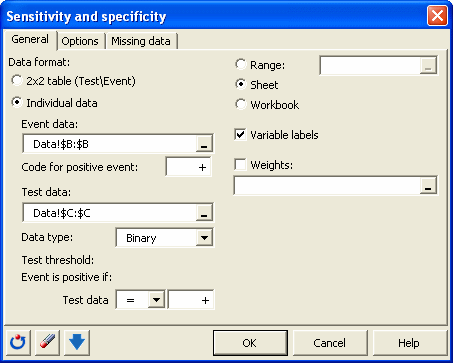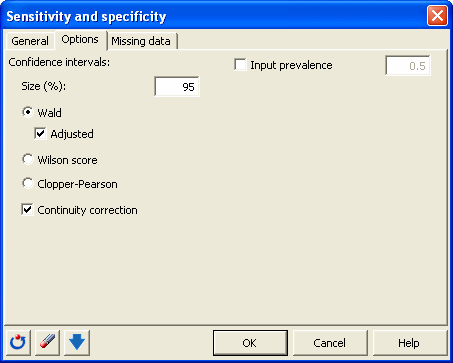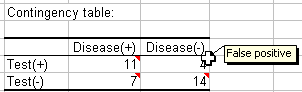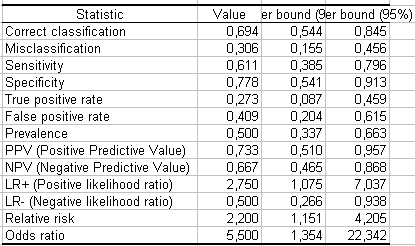# Sensitivity and specificity in Excel tutorial

2017-11-17

This tutorial will show you how to set up and interpret a sensitivity and specificity analysis in Excel using the XLSTAT software.

## Sensitivity and specificity analysis

This method was first introduced during World War II to develop effective means of detecting Japanese aircrafts. It was then applied more generally to signal detection and medicine where it is now widely used.

The problem is as follows: we study a phenomenon, often binary (for example, the presence or absence of a disease) and we want to develop a test to detect effectively the occurrence of a precise event (for example, the presence of the disease).

Let V be the binary or multinomial variable that describes the phenomenon for N individuals that are being followed. We note by + the individuals for which the event occurs and by -those for which it does not. Let T be a test which goal is to detect if the event occurred or not. T can be a binary (presence/absence), a qualitative (for example the color), or a quantitative variable (for example a concentration).

Once the test has been applied to the N individuals, we get an individuals/variables table where for each individual the occurrence of the event, and the result of test are recorded.

## Dataset for sensitivity and specificity analysis

An Excel sheet with both the data and results used in this tutorial can be downloaded by clicking here.

The data correspond to a medical experiment during which 18 patients with a disease and 18 healthy individuals have been submitted to a new diagnostic test, less expensive than the current very powerful one. This test is binary, as it is supposed to show a red color when the patient is sick and no color in the opposit case.

The results are recorded in an individuals/variables table . We want to use a sensitivity and specificity analysis to evaluate the test.

## Setting up a sensitivity and specificity analysis

Once XLSTAT has been started, select the Survival analysis / Sensitivity and specificity command.When you click on the button, a dialog box appears. Select the data that correspond to the event data and to the test data and enter which code is associated to positive cases for both data sets.In the Options tab, you can specify the method for calculating the confidence intervals. XLSTAT is the software offering the widest choice. The defaults are those most recommended.When you click OK, the computations are done and the results are displayed.

## interpretation of the results on a sensitivity and specificity analysis

The first table is a contingency table (crosstab) that summarizes the input table with the following values:

• True positive’(TP): Number of cases that the test declares positive and that are truly positive.
• False positive (FP): Number of cases that the test declares positive and that in reality are negative.
• True negative (VN): Number of cases that the test declares negative and that are truly negative.
• False negative (FN): Number of cases that the test declares negative and that in reality are positive.Using these counts and N the sum of these values, we compute the various indices that allow evaluating the performance of the diagnostic test:To ease the interpretation of these results, here is a description of the various indices:

• Sensitivity (equivalent to the True Positive Rate): Proportion of positive cases that are well detected by the test. In other words, the sensitivity measures how the test is effective when used on positive individuals. The test is perfect for positive individuals when sensitivity is 1, equivalent to a random draw when sensitivity is 0.5. If it is below 0.5, the test is counter-performing and it would be useful to reverse the rule so that sensitivity is higher than 0.5 (provided that this does not affect the specificity). The mathematical definition is given by: Sensitivity = TP/(TP + FN).
• Specificity (also called True Negative Rate): proportion of negative cases that are well detected by the test. In other words, specificity measures how the test is effective when used on negative individuals. The test is perfect for negative individuals when the specificity is 1, equivalent to a random draw when the specificity is 0.5. If it is below 0.5, the test is counter performing-and it would be useful to reverse the rule so that specificity is higher than 0.5 (provided that this does not affect the sensitivity). The mathematical definition is given by: Specificity = TN/(TN + FP).
• False Positive Rate (FPR): Proportion of negative cases that the test detects as positive (FPR = 1-Spécificité).
• False Negative Rate (FNR): Proportion of positive cases that the test detects as negative (FNR = 1-Sensibilité)
• Prevalence: relative frequency of the event of interest in the total sample (TP+FN)/N.
• Positive Predictive Value (PPV): Proportion of truly positive cases among the positive cases detected by the test. We have PPV = TP / (TP + FP), or PPV = Sensitivity x Prevalence / [(Sensitivity x Prevalence + (1-Specificity)(1-Prevalence)]. It is a fundamental value that depends on the prevalence, an index that is independent of the quality of the test.
• Negative Predictive Value (NPV): Proportion of truly negative cases among the negative cases detected by the test. We have NPV = TN / (TN + FN), or PPV = Specificity x (1 - Prevalence) / [(Specificity (1-Prevalence) + (1-Sensibility) x Prevalence]. This index depends also on the prevalence that is independent of the quality of the test.
• Positive Likelihood Ratio (LR+): This ratio indicates to which point an individual has more chances to be positive in reality when the test is telling it is positive. We have LR+ = Sensitivity / (1-Specificity). The LR+ is a positive or null value.
• Negative Likelihood Ratio (LR-): This ratio indicates to which point an individual has more chances to be negative in reality when the test is telling it is positive. We have LR- = (1-Sensitivity) / (Specificity). The LR- is a positive or null value.
• Odds ratio: The odds ratio indicates how much an individual is more likely to be positive if the test is positive, compared to cases where the test is negative. For example, an odds ratio of 2 means that the chance that the positive event occurs is twice higher if the test is positive than if it is negative. The odds ratio is a positive or null value. We have Odds ratio = TPxTN / (FPxFN).
• Relative risk: The relative risk is a ratio that measures how better the test behaves when it is a positive report than when it is negative. For example, a relative risk of 2 means that the test is twice more powerful when it is positive that when it is negative. A value close to 1 corresponds to a case of independence between the rows and columns, and to a test that performs as well when it is positive as when it is negative. Relative risk is a null or positive value given by: Relative risk = TP/(TP+FP) / (FN/(FN+TN)).

The performance of the test is pretty average and neither the sensitivity nor the specificity are really satisfactory. However, the very low cost of the test makes it interesting. A slight improvement in the sensitivity and a linkage with another test could make it effective.

Note: The predictive values are biased in this case. Indeed, the prevalence of the disease in our sample is 50% (1 person over 2 is ill), which does not correspond to the reality of the total population where the disease affects one person in 2000. To correct the predictive values, you only need in the "Options" tab to indicate that the input prevalence is 0.0005.

#### Contact our technical support team: support@xlstat.com

https://cdn.desk.com/
false
desk